## 设计资源

• 原理图
• 物料清单
• Gerber 文件
• 装配图

### 评估硬件

• EVAL-CN0289-EB1Z (\$80.00) Flexible, 4 mA-to-20 mA, Loop-Powered Pressure Sensor Transmitter with Voltage or Current Drive

## 优势和特点

• 4-20mA环路供电发射器
• 电压或电流驱动
• 优化压力传感器

## 电路描述

 元件 电流(mA) ADR02 0.80 ADA4091-2 0.50 AD8226 0.43 R5, R6 @ 10V 0.05 R12 @ 5V 0.04 TOTAL 1.82

### 激励：电压驱动配置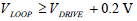### 激励：电流驱动配置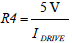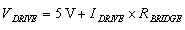U2A电源需要0.2 V裕量，因此：### 电桥输出仪表放大器以及增益和失调电阻选择

AD8226是理想的仪表放大器选择，因为它具有低增益误差(0.1%，B级)、低失调(G = 50时58μV，B级；G = 50时112μV，A级)、出色的增益非线性度(75 ppm = 0.0075%)以及轨到轨输出特性。

AD8226仪表放大器以系数50 V至5 V放大100 mV FS信号，增益设置电阻R3 = 1.008 kΩ。增益G和R3的关系如下：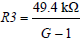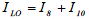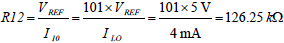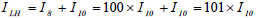R9值可通过下式计算：• R3 = 1 kΩ + 8.06 Ω = 1008.06 Ω (计算值 = 1008 Ω)
• R9 = 30.9 kΩ + 655 Ω = 31.555k Ω (计算值 = 31.56 kΩ)
• R12 = 124 kΩ + 2.26 kΩ = 126.26 Ω (计算值 = 126.25 Ω)

• 失调误差 = −0.008% FSR
• 增益误差 = +0.010% FSR
• 满量程误差 =

• R3 = 1000 Ω(计算值 = 1008 Ω)
• R9 = 31.6 kΩ(计算值 = 31.56 kΩ)
• R12 = 124 kΩ(计算值 = 126.25 kΩ)

• 失调误差 = +0.45% FSR
• 增益误差 = +0.66% FSR
• 满量程误差 = +1.11% FSR

### 基准电压### 总电路精度

• 失调误差 = 0.29% +0.5% = 0.79%
• 增益误差 = 0.15% + 0.5% = 0.65%
• 满量程误差 = 0.44% + 0.5% = 0.94%

## 电路评估与测试

### 设备要求

• EVAL-CN0289-EB1Z评估板
• Agilent E36311A双直流电源(或等效设备)
• Agilent 3458A万用表(或等效设备)

### 电流输出测量

• 环路电源：24 V
• 环路负载：250 Ω
• RBRIDGE = 3 kΩ
• VDRIVE = 5 V
• VCM = 2.5 V

### 测试设置的配置与测试

Agilent 3458A用于测量评估板的实际环路电流输出。